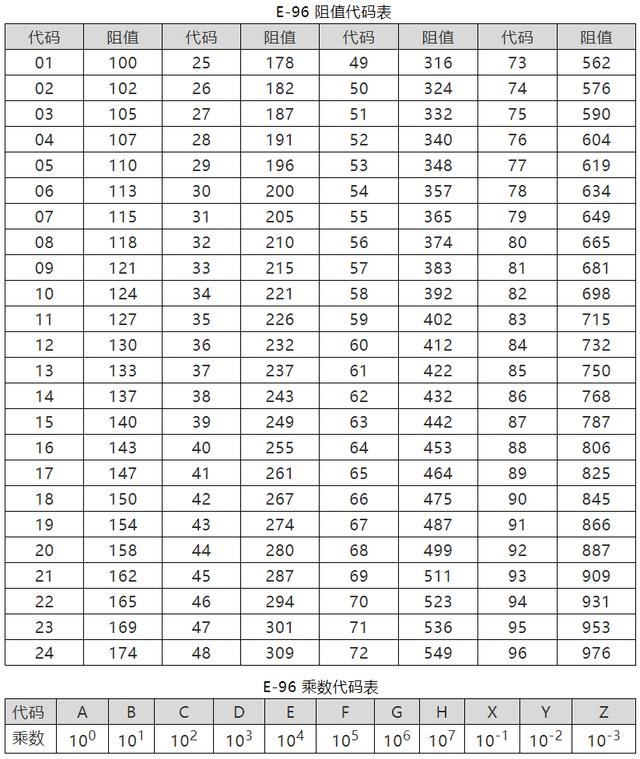# 基本元器件 - 电阻

## 电阻的选型

• 阻值：根据具体应用电路的需要而定
• 精度：通常为 1%，如果用于电流检测回路（Rsense），通常低阻值大功率更高精度
• 额定功率：满足 50% 降额，不同封装对应功率请见下表
• 尺寸：尺寸与功率相关，应考虑功率和加工难度而定
• 工作温度、湿度等：特定时候需要考虑的因素
• 温漂：如果用于高精度（传感器应用），则必须考虑

## 贴片封装的参数

0201 0603 0.60±0.05 0.30±0.05 0.23±0.05 1/20 25
0402 1005 1.00±0.10 0.50±0.10 0.30±0.10 1/16 50
0603 1608 1.60±0.15 0.80±0.15 0.40±0.10 1/10 50
0805 2012 2.00±0.20 1.25±0.15 0.50±0.10 1/8 150
1206 3216 3.20±0.20 1.60±0.15 0.55±0.10 1/4 200
1210 3225 3.20±0.20 2.50±0.20 0.55±0.10 1/3 200
1812 4832 4.50±0.20 3.20±0.20 0.55±0.10 1/2 200
2010 5025 5.00±0.20 2.50±0.20 0.55±0.10 3/4 200
2512 6432 6.40±0.20 3.20±0.20 0.55±0.10 1 200

## 电阻的阻值

### 丝印表示方法

• 三位数标注法$$XXY = XX * 10^Y$$
• 例如，丝印为 272 的电阻，实际阻值为 $$27 * 10^2=27 * 100=2.7k$$
• 四位数标注法$$XXXY = XXX * 10^Y$$
• 字母表示小数点位置法R 表示小数点。
• 例如，丝印为 5R6 的电阻，实际阻值为 5.6 Ω
• M k m 也都可以表示小数点，分别代表 MΩ kΩ mΩ
• 三位数乘数代码标注法XXY 中，XX 表示有效数的代码，Y 指是 10 的多少次幂，可参考下方的标准电阻取值表
• 例如，丝印为 01C 的电阻，实际阻值为 $$100*10^2=10 kΩ$$

### 标准电阻取值## 电阻的失效

• 开路：电阻膜缺陷或退化；瞬时功率过大冲击时可能导致
• 阻值漂移超规范：老化后有可能发生
• 引脚断裂：焊接工艺缺陷、焊点污染；插件电阻引脚反复弯曲时可能发生
• 烧毁：长时间工作在额定功率以上，有可能烧毁导致开路
• 焊接问题：虚焊等问题
• 断线开路：受机械应力或瞬时过功率冲击时可能发生

## 0 欧姆电阻的使用

• 当跳线用，跨过布不下线的区域
• 作为短接座用
• 单点连接数字地和模拟地（有时也用电感或磁珠）
• 预留阻值用于调试

0201 0.5（1）
0402 1（2）
0603 2（3）
0805 及以上 2（5）

## 电阻的使用场景

### 分压电路

• 通过各电阻的电流是同一电流，即各电阻中的电流相等，即 $$I = I_1 = I_2 = I_3$$
• 总电压等于各电阻上的电压降之和，即 $$V= V_1 + V_2 + V_3$$
• 总电阻等于各电阻之和，即 $$R=R_1 + R_2 +R_3$$

### 分流电路

• 各支路两端电压相等
• 总电流等于各支路电流之和，即 $$I= I_1 + I_2 + I_3$$
• 总电阻的倒数等于各支路倒数之和，即 $$\frac{1}{R}=\frac{1}{R_1}+\frac{1}{R_2}+\frac{1}{R_3}$$

### 阻抗匹配电路

$I=\frac{U}{R+r}$

$U_R=IR=\frac{U}{1+\frac{r}{R}}$

R 越大，则输出电压 $$U_R$$ 越大。R 的功率为：

$P=I^2R=(\frac{U}{R+r})^2R=\frac{U^2R}{R^2+r^2+2Rr}=\frac{U^2}{\frac{(R-r)^2}{R}+\frac{4Rr}{R}}$

### RC 充放电电路

$$\tau=RC$$（若 R 和 C 的单位为 Ω 和 F，则结果的单位为 s。

RC 电路可视为延时电路或滤波电路，将脉冲信号上升下降沿都进行了滤波，使其变得平缓，可以通过调整 R、C 值，以实现不同上升时间。

### 其他电路

• 运算放大器外围电路
• 抗干扰电路，提高抗浪涌电压能力
• 负载电路（防止电路空载）